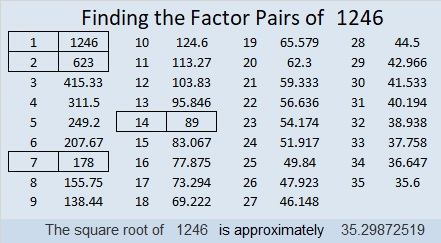# 1246 and Level 4

The reason level 4 puzzles are more difficult than level 3 puzzle is that you have to look all over the puzzle to find the next clue that will help you solve it. Still, there are only 10 clues in this puzzle, so you don’t have to look in very many places. Go ahead and give this puzzle a try!Print the puzzles or type the solution in this excel file: 10-factors-1242-1250

Let me share some facts about the number 1246:

• 1246 is a composite number.
• Prime factorization: 1246 = 2 × 7 × 89
• The exponents in the prime factorization are 1, 1, and 1. Adding one to each and multiplying we get (1 + 1)(1 + 1)(1 + 1) = 2 × 2 × 2 = 8. Therefore 1246 has exactly 8 factors.
• Factors of 1246: 1, 2, 7, 14, 89, 178, 623, 1246
• Factor pairs: 1246 = 1 × 1246, 2 × 623, 7 × 178, or 14 × 89
• 1246 has no square factors that allow its square root to be simplified. √1246 ≈ 35.298731246 is the hypotenuse of a Pythagorean triple:
546-1120-1246 which is 14 times (39-80-89)

This site uses Akismet to reduce spam. Learn how your comment data is processed.